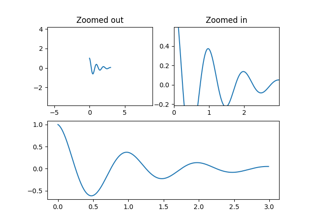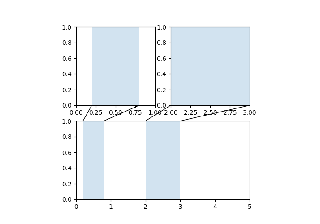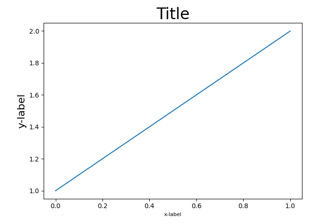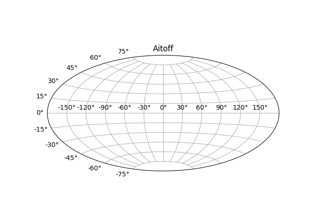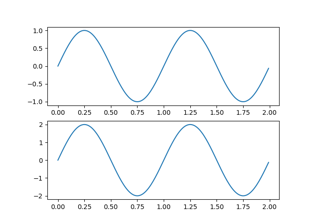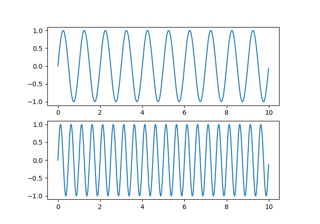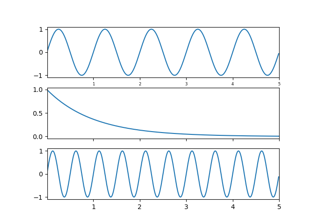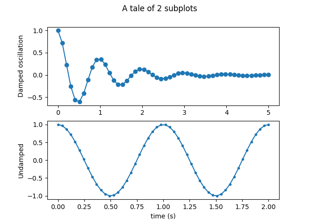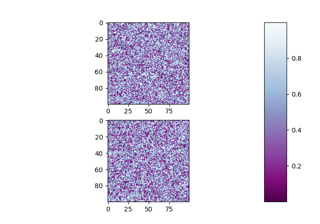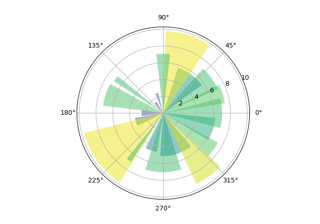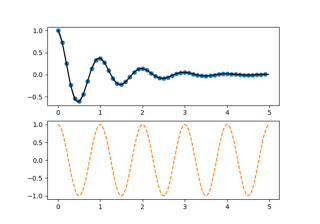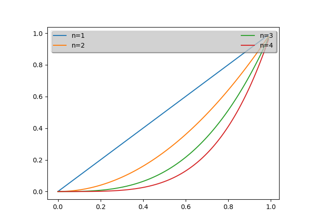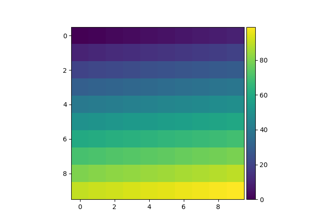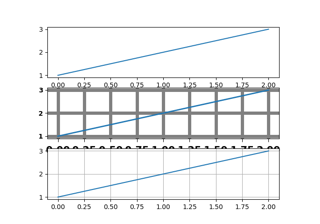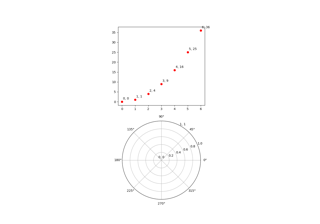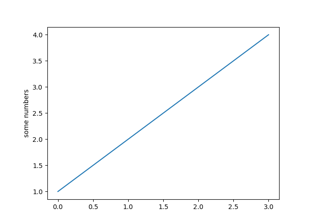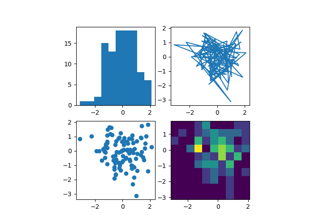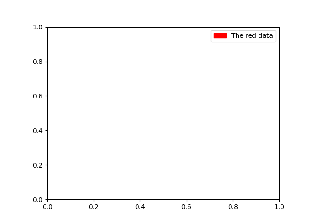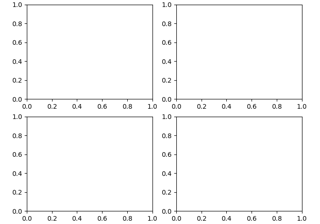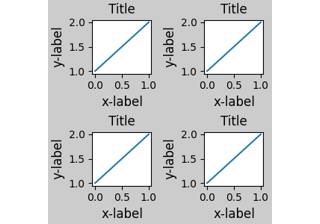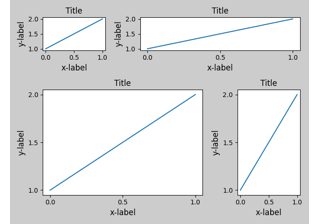# matplotlib.pyplot.subplot¶

matplotlib.pyplot.subplot(*args, **kwargs)[source]

Add an Axes to the current figure or retrieve an existing Axes.

This is a wrapper of Figure.add_subplot which provides additional behavior when working with the implicit API (see the notes section).

Call signatures:

subplot(nrows, ncols, index, **kwargs)
subplot(pos, **kwargs)
subplot(**kwargs)
subplot(ax)

Parameters:
*argsint, (int, int, index), or SubplotSpec, default: (1, 1, 1)

The position of the subplot described by one of

• Three integers (nrows, ncols, index). The subplot will take the index position on a grid with nrows rows and ncols columns. index starts at 1 in the upper left corner and increases to the right. index can also be a two-tuple specifying the (first, last) indices (1-based, and including last) of the subplot, e.g., fig.add_subplot(3, 1, (1, 2)) makes a subplot that spans the upper 2/3 of the figure.
• A 3-digit integer. The digits are interpreted as if given separately as three single-digit integers, i.e. fig.add_subplot(235) is the same as fig.add_subplot(2, 3, 5). Note that this can only be used if there are no more than 9 subplots.
• A SubplotSpec.
projection{None, 'aitoff', 'hammer', 'lambert', 'mollweide', 'polar', 'rectilinear', str}, optional

The projection type of the subplot (Axes). str is the name of a custom projection, see projections. The default None results in a 'rectilinear' projection.

polarbool, default: False

If True, equivalent to projection='polar'.

sharex, shareyAxes, optional

Share the x or y axis with sharex and/or sharey. The axis will have the same limits, ticks, and scale as the axis of the shared axes.

labelstr

A label for the returned axes.

Returns:
axes.SubplotBase, or another subclass of Axes

The axes of the subplot. The returned axes base class depends on the projection used. It is Axes if rectilinear projection is used and projections.polar.PolarAxes if polar projection is used. The returned axes is then a subplot subclass of the base class.

Other Parameters:
**kwargs

This method also takes the keyword arguments for the returned axes base class; except for the figure argument. The keyword arguments for the rectilinear base class Axes can be found in the following table but there might also be other keyword arguments if another projection is used.

Property Description
adjustable {'box', 'datalim'}
agg_filter a filter function, which takes a (m, n, 3) float array and a dpi value, and returns a (m, n, 3) array
alpha scalar or None
anchor 2-tuple of floats or {'C', 'SW', 'S', 'SE', ...}
animated bool
aspect {'auto'} or num
autoscale_on bool
autoscalex_on bool
autoscaley_on bool
axes_locator Callable[[Axes, Renderer], Bbox]
axisbelow bool or 'line'
box_aspect float or None
clip_box Bbox
clip_on bool
clip_path Patch or (Path, Transform) or None
contains unknown
facecolor or fc color
figure Figure
frame_on bool
gid str
in_layout bool
label object
navigate bool
navigate_mode unknown
path_effects AbstractPathEffect
picker None or bool or float or callable
position [left, bottom, width, height] or Bbox
prop_cycle unknown
rasterization_zorder float or None
rasterized bool
sketch_params (scale: float, length: float, randomness: float)
snap bool or None
title str
transform Transform
url str
visible bool
xbound unknown
xlabel str
xlim (bottom: float, top: float)
xmargin float greater than -0.5
xscale {"linear", "log", "symlog", "logit", ...} or ScaleBase
xticklabels unknown
xticks unknown
ybound unknown
ylabel str
ylim (bottom: float, top: float)
ymargin float greater than -0.5
yscale {"linear", "log", "symlog", "logit", ...} or ScaleBase
yticklabels unknown
yticks unknown
zorder float

Notes

Creating a new Axes will delete any pre-existing Axes that overlaps with it beyond sharing a boundary:

import matplotlib.pyplot as plt
# plot a line, implicitly creating a subplot(111)
plt.plot([1, 2, 3])
# now create a subplot which represents the top plot of a grid
# with 2 rows and 1 column. Since this subplot will overlap the
# first, the plot (and its axes) previously created, will be removed
plt.subplot(211)


If you do not want this behavior, use the Figure.add_subplot method or the pyplot.axes function instead.

If no kwargs are passed and there exists an Axes in the location specified by args then that Axes will be returned rather than a new Axes being created.

If kwargs are passed and there exists an Axes in the location specified by args, the projection type is the same, and the kwargs match with the existing Axes, then the existing Axes is returned. Otherwise a new Axes is created with the specified parameters. We save a reference to the kwargs which we use for this comparison. If any of the values in kwargs are mutable we will not detect the case where they are mutated. In these cases we suggest using Figure.add_subplot and the explicit Axes API rather than the implicit pyplot API.

Examples

plt.subplot(221)

# equivalent but more general
ax1 = plt.subplot(2, 2, 1)

# add a subplot with no frame
ax2 = plt.subplot(222, frameon=False)

plt.subplot(223, projection='polar')

# add a red subplot that shares the x-axis with ax1
plt.subplot(224, sharex=ax1, facecolor='red')

# delete ax2 from the figure
plt.delaxes(ax2)

# add ax2 to the figure again
plt.subplot(ax2)

# make the first axes "current" again
plt.subplot(221)


## Examples using matplotlib.pyplot.subplot¶